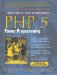# Section 3.11. instanceof Operator

### 3.11. instanceof Operator

The instanceof operator was added as syntactic sugar instead of the already existing is_a() built-in function (which is now deprecated). Unlike the latter, instanceof is used like a logical binary operator:

` class Rectangle {     public \$name = __CLASS__; } class Square extends Rectangle {     public \$name = __CLASS__; } class Circle {     public \$name = __CLASS__; } function checkIfRectangle(\$shape) {     if (\$shape instanceof Rectangle) {         print \$shape->name;         print " is a rectangle\n";     } } checkIfRectangle(new Square()); checkIfRectangle(new Circle()); `

This small program prints 'Square is a rectangle\n'. Note the use of __CLASS__, which is a special constant that resolves to the name of the current class.

As previously mentioned, instanceof is an operator and therefore can be used in expressions in conjunction to other operators (for example, the ! [negation] operator). This allows you to easily write a checkIfNotRectangle() function:

` function checkIfNotRectangle(\$shape) {     if (!(\$shape instanceof Rectangle)) {         print \$shape->name;         print " is not a rectangle\n";     } } `

Note

instanceof also checks if an object implements an interface (which is also a classic is-a relationship). Interfaces are covered later in this chapter.PHP 5 Power Programming
ISBN: 013147149X
EAN: 2147483647
Year: 2003
Pages: 240

Similar book on Amazon